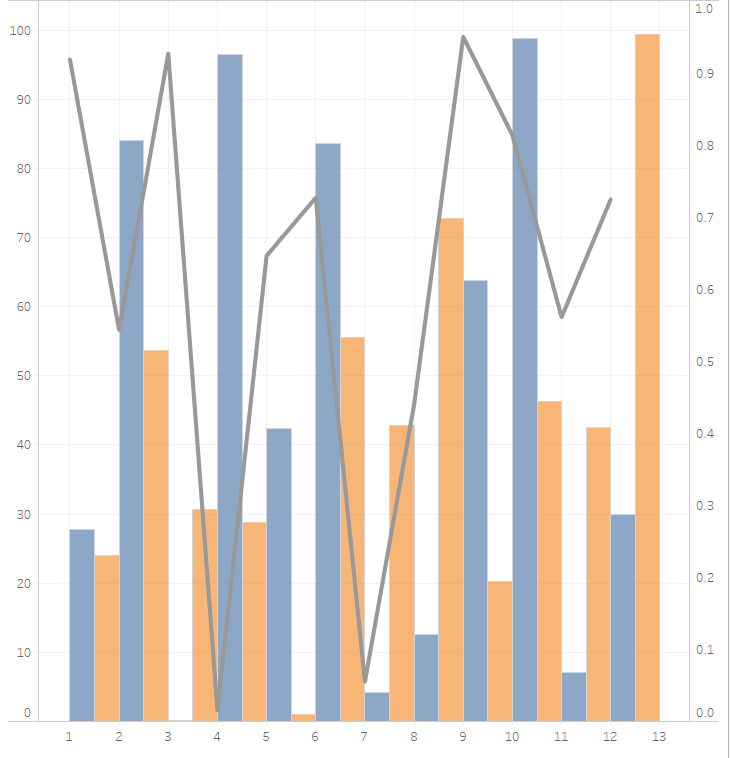## Blog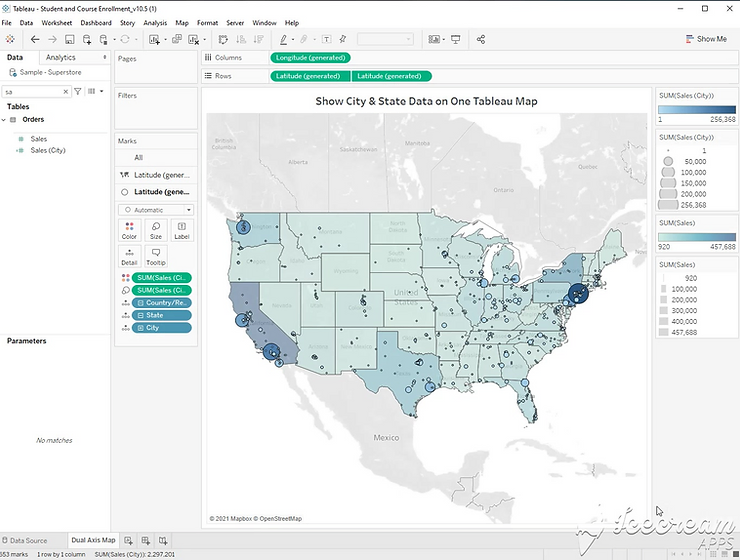Blogs### Show City & State Data on One Tableau Map

Step 1: Double Click [State] to create a map. Double click [City] Step 2: Right click [Sales] and create a calculated field. You will need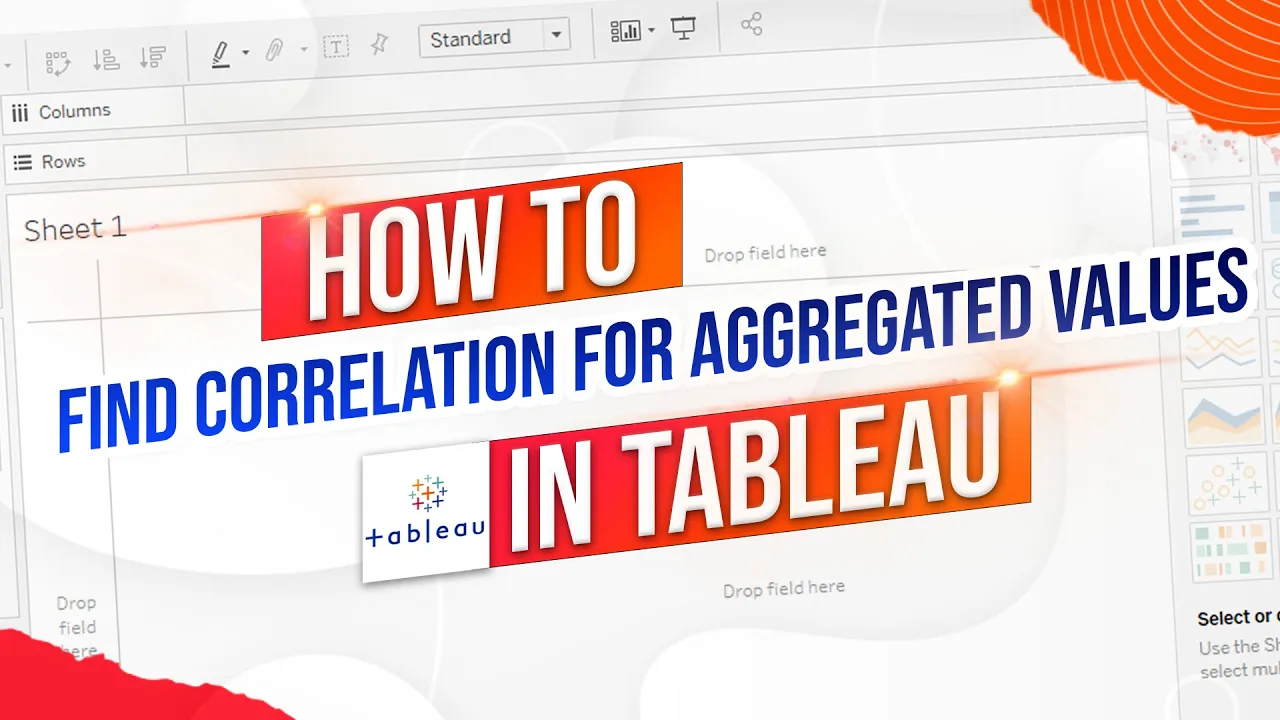Blogs### How to Find the Correlation for an Aggregated Value in Tableau Using a Window Function

How do you find the correlation of two aggregated values in tableau in this example I have a scatter plot of kpi and kpi2 and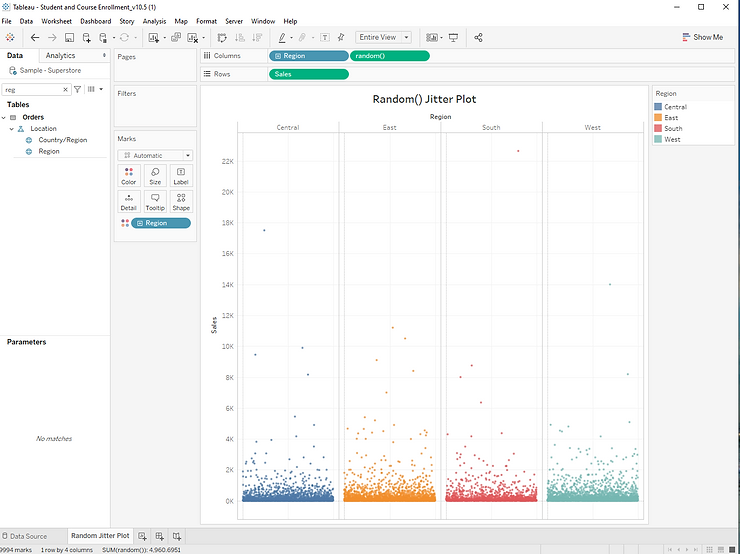Blogs### Tableau: RANDOM() Jitter Plot

Step 1: Drag [Sales] to the rows shelf, and [Region] to the columns shelf. Step 2: Click Analysis, and unselect “Aggregate Measures”. This will result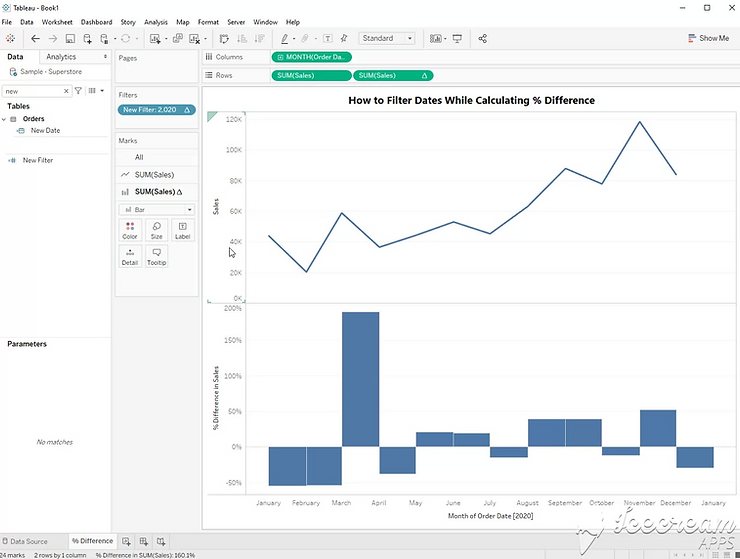Blogs### Tableau: How to Filter Dates While Calculating % Difference

Filters can cause problems when using table calculations in Tableau. Here’s a trick to use a filter while also using table calculations. We’ll use the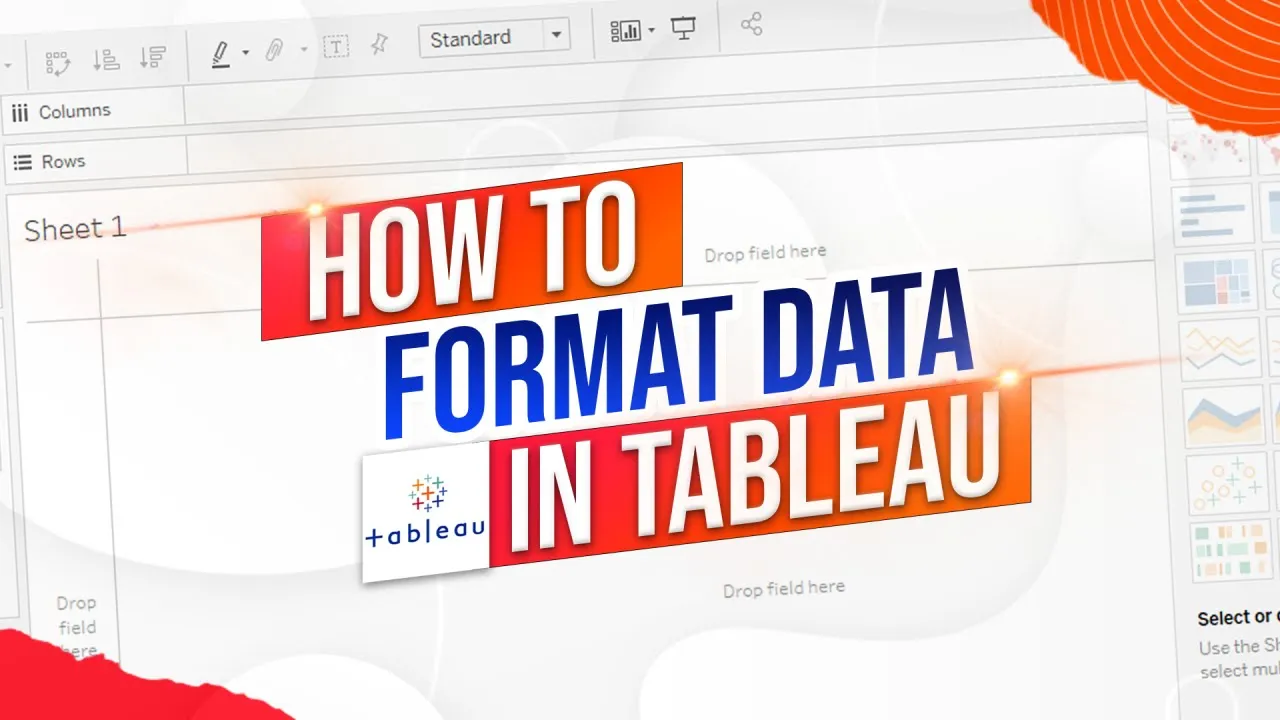Blogs### How to Format Positive and Negative Values in Tableau Using Color and Custom Formats

How do you format positive and negative values in tableau in this example I have sum of profit by state and region and I’m showing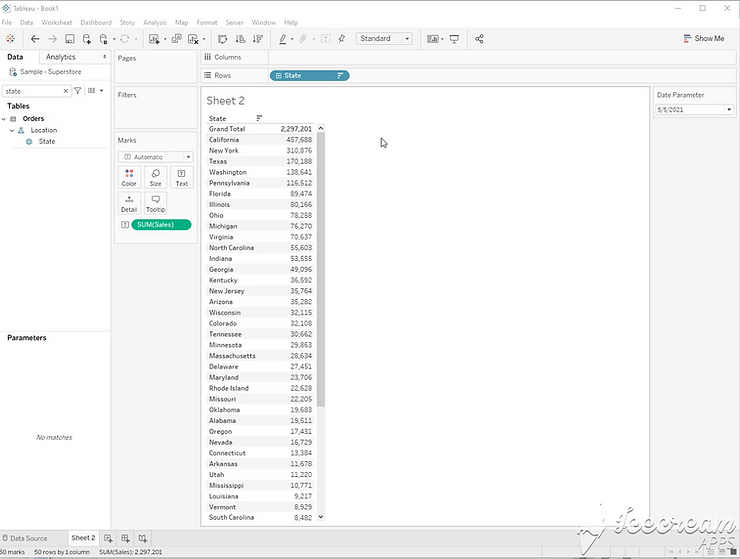Blogs### Tableau: How to Display Correct Grand Total with a Rank Filter

Step 1: Start with [State] on rows, and sum([Sales]) on Text. Step 2: Create a rank calculation. Rank(Sum([Sales])) Step 3: Add the calculation to the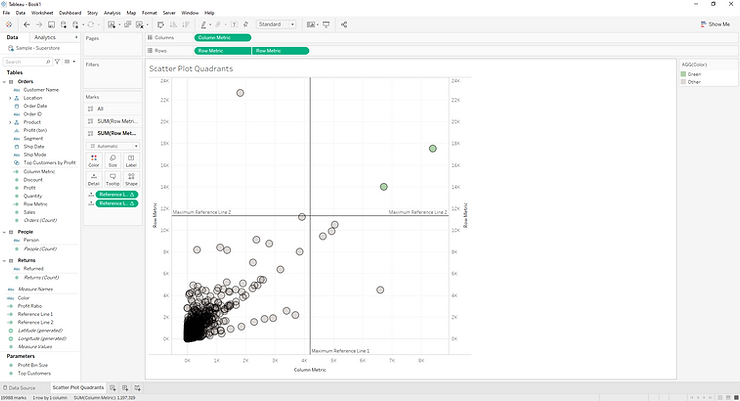BlogsStep 1: Build a scatter Plot using a metric on columns and a metric on rows. Unselect aggregated measures to finalize the scatter plot. Step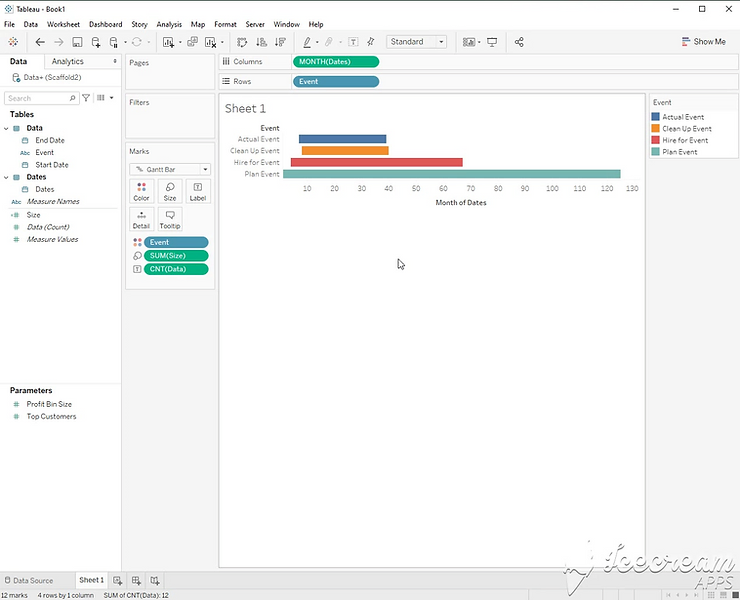Blogs### Tableau: Create a Gantt Chart using Start & End Dates (Scaffold)

Step 1: Create a Dates Table Step 2: Join the dates table with your data on [Start Date] <= [Date] and [End Date] >= [Date]Blogs### Tableau: How to Solve “Cannot Mix Aggregate and Non-Aggregate” Errors

Let’s start with a simple example. Within a single calculation, all parts of a calculation must be either aggregated or not aggregated. This calculation is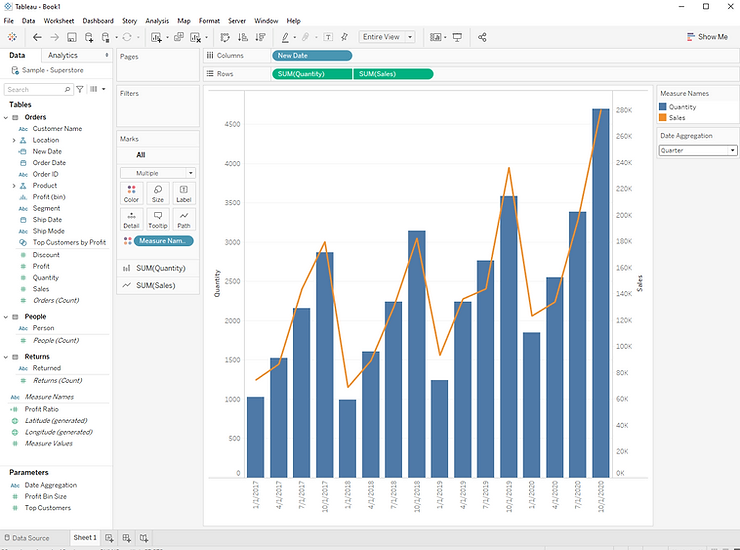Blogs### Tableau: Control Date Level with Parameters (Year, Month, Quarter)

Step 1: Build a dual axis chart using [Date], [Quantity], and [Sales]. The result will be a combined bar and line chart. Step 2: Create